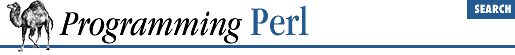home | O'Reilly's CD bookshelfs | FreeBSD | Linux | Cisco | Cisco Exam

#### 7.2.69 Text::Soundex - The Soundex Algorithm Described by Knuth

```use Text::Soundex;

\$code = soundex \$string;  # get soundex code for a string
@codes = soundex @list;   # get list of codes for list of strings

# set value to be returned for strings without soundex code
\$soundex_nocode = 'Z000';```

This module implements the soundex algorithm as described by Donald Knuth in Volume 3 of The Art of Computer Programming . The algorithm is intended to hash words (in particular surnames) into a small space using a simple model that approximates the sound of the word when spoken by an English speaker. Each word is reduced to a four-character string, the first character being an uppercase letter and the remaining three being digits.

If there is no soundex code representation for a string, then the value of ``` \$soundex_nocode``` is returned. This variable is initially set to the undefined value, but many people seem to prefer an unlikely value like ``` Z000``` . (How unlikely this is depends on the data set being dealt with.) Any value can be assigned to ``` \$soundex_nocode``` .

In a scalar context ``` soundex()``` returns the soundex code of its first argument, and in an array context a list is returned in which each element is the soundex code for the corresponding argument passed to ``` soundex()``` .

For example:

`@codes = soundex qw(Mike Stok);`

leaves ``` @codes``` containing ``` ('M200', 'S320')``` .

Here are Knuth's examples of various names and the soundex codes they map to:

Names Code
Euler, Ellery ``` E460```
Gauss, Ghosh ``` G200```
Hilbert, Heilbronn ``` H416```
Knuth, Kant ``` K530```
Lloyd, Ladd ``` L300```
Lukasiewicz, Lissajous ``` L222```

So we have:

```\$code = soundex 'Knuth';              # \$code contains 'K530'
@list = soundex qw(Lloyd Gauss);      # @list contains 'L300', 'G200'```

As the soundex algorithm was originally used a long time ago in the United States, it considers only the English alphabet and pronunciation.

As it is mapping a large space (arbitrary-length strings) onto a small space (single letter plus three digits), no inference can be made about the similarity of two strings that end up with the same soundex code. For example, both ``` Hilbert``` and ``` Heilbronn``` end up with a soundex code of ``` H416``` .7.2.68 Text::ParseWords - Parse Text into a List of Tokens7.2.70 Text::Tabs - Expand and Unexpand Tabs